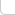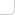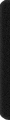# 某岛

… : "…アッカリ～ン . .. . " .. .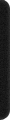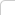October 21, 2021

## Facebook Hacker Cup 2021 Round 1

A1.

A2

const int N = int(1e6) + 9;
char s[N];
int n;

int main() {

#ifndef ONLINE_JUDGE
freopen("in.txt","r",stdin);
freopen("out.txt","w",stdout);
#endif

Rush {
RD(n); RS(s);
Int z = 0;
char lc = '?'; // 上一个字符
int lp = 0; // 上一个字符的位置

REP(i, n) {
char c = s[i];
if(c != 'F') {
if (lc != c) {
if(lc != '?') z += Int(lp) * (n-i);
lc = c;
}
lp = i+1;
}
}
OT(z);
}
}


A3
s 中还包含 ‘.’ 字符，如果出现 ‘.’ 字符，则用此前的字符串替换 ‘.’ 位置，询问 A2。

const int N = int(1e6) + 9;
char s[N];
int n;

int main() {

#ifndef ONLINE_JUDGE
freopen("in.txt","r",stdin);
freopen("out.txt","w",stdout);
#endif

Rush {
RD(n); RS(s);
Int z = 0;
char lc = '?'; // 上一个字符
int lp = 0; // 上一个字符的位置
Int ls = 0; // 右边新增加一个字符时答案的增量。

REP(i, n) {
z += ls;
char c = s[i];
if (c != 'F') {
if (lc != '?' && lc != c) {
z += lp;
ls += lp;
}
lp = i+1;
lc = c;
}
}
OT(z);
}
}


const int N = int(1e6) + 9;
char s[N];
int n;

int main() {

#ifndef ONLINE_JUDGE
freopen("in.txt","r",stdin);
freopen("out.txt","w",stdout);
#endif

Rush {
RD(n); RS(s);
Int z = 0;
char lc = '?', rc = '?'; //
Int lp = 0, rp = 0, ct = 0;
Int ls = 0, rs = 0;
Int len = 0;

REP(i, n) {
char c = s[i];
if (c == '.') {

z = z*2 + len*(ls+rs);
ls = ls*2 + len*ct;
rs = rs*2 + len*ct;
ct *= 2;

if (lc != rc) {
ct += 1; ls += lp; rs += (len-rp+1);
z += lp * (len-rp+1);
}
if (lc != '?') lp += len;
len *= 2;

} else {

z += ls;
len += 1;

if (c != 'F') {

if (rc == '?') {
rc = c, rp = len;
}

if (lc != '?' && lc != c) {
z += lp;
ls += lp;
ct += 1;
}
lp = len;
lc = c;
}

rs += ct;
}
}
OT(z);
}
}


C.